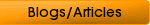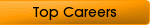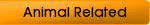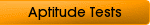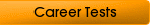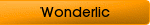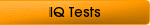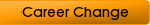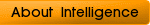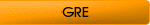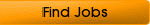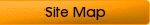## Passing a Math Aptitude Test

More and more employers are using a math aptitude test to test the basic skills of prospective employees. While you may not need to be brilliant at maths for most jobs, a computer scientist or an engineer will need good maths skills.

Maths may not be your strong point, but most organizations want to know just what your mathematical ability is before they consider hiring you. Different organizations use different types of math aptitude tests for different roles, and will include questions from some of the following areas:

• profit and loss

• statistics

• algebra

• geometry

• probability

• trigonometry

• number series

• permutations and combinations

### Importance of Algebra

Algebra is the basis of almost all math aptitude tests. Employers use them to determine your overall understanding of mathematical principles and your ability to put it into practice.Aptitude test questions are meant to discover what your achievements fail to tell employers. They answer a lot of questions a prospective employer may have, such as:

• Are you capable of identifying an equation to solve written problems?

• Do you understand algebraic symbols and how to use them?

• What is your ability to interpret algebraic expressions?

• What is your capacity to learn algebra quickly and efficiently?

• Can you see relationships between sets of numbers?

### Understanding Mathematical Symbols

Before you take an aptitude test you need to understand the basic symbols. Here are a few and what they mean:

• √ means the square root of something (√25 = 5)

• |x| means the absolute value

• ≤ means it is lesser than or equal to

• ≥ means it is greater than or equal to

• > means it is greater than (132 > 99)

• < means it is lesser than (99 < 132)

• ≠ means it is not equal to

• : and :: means to the ratio to

### Types of Algebraic Problems

Numerical tests have questions from a cross section of mathematical disciplines such as the roman system, measurements, and geometry. The point of aptitude test questions is to measure your ability to use your mathematical skills to solve problems.

You will need to identify the underlying principles and any relationships within mathematical problems to answer them correctly. Math aptitude tests tend to test your analytical skills rather than that you can add up.These tests will include questions from some of the following mathematical skills:

• greatest common divisor

• least common divisor

• factoring the difference of squares

• factoring out a common divisor

• factoring the squares of binomials

• evaluation of an expression

• exponents

• trinomial multiplication

• monomial multiplication

• binomial multiplication

• algebraic fraction simplification

• square root of an algebraic expression

### What is the Roman System?

When using the Roman system the numbers are represented by Roman numerals such as D for 500 and ix for nine. If a smaller number is on the left it indicates you need to subtract, and if it is to the right you need to add.

These questions test your basic understanding of geometry, and its principles and uses to help problem solve. You need to have basic knowledge of:

• the terminology and characteristics of angles

• circles

• ordinate numbers

• the concepts of polygon

• perimeters

• hexagons

• the rectangular coordinator system

• pentagons

Before encountering a math aptitude test, you are wise to brush up on some sample tests to test just how well you do.

### Questions Involving Measurements

Nearly every math aptitude test has questions involving measurements in some way. You need to use different techniques such as weight, space, and time to solve the problems.

### Statistical and Probability Data

Many jobs require some sort of statistical analysis and most math aptitude tests will determine your ability to analyse situations and reach a positive outcome using your math reasoning skills. Before sitting an aptitude test, brush up on how to collect and analyse organizational statistical data and how to interpret what it means.

Home
Quantitative Aptitude Test
Numerical Test
Algebra Test
Careers In Math
Numerical Aptitude Tests
General Aptitude Test Battery